# Execution Recovery Examples and Formulations

This page contains full formulations and more complete examples for the paper ‘‘Recovering Execution Data from Incomplete Observations,’’ published in the 13th International Workshop on Dynamic Analysis (WODA 2015).

## Example Problem

The example problem (from the WODA paper) is as follows. First, we are given the following control-flow graph (CFG):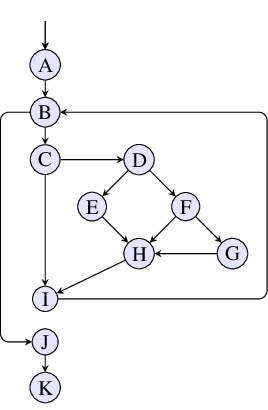The remainder of our failure report is as follows: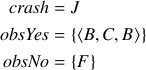The goal of problem is to compute three sets: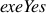(containing those nodes that occur on all paths through our CFG consistent with the failure report),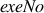(containing those nodes that occur on no paths matching the failure report), and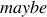(containing those nodes that occur on some but not all such paths). The following two sections provide the full formulations to solving this problem that we describe in the WODA paper.

## Finite-State Automata

This section provides the full collection of automata used for Section 2.1 of the paper.

### Automata for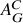The first collection of automata encode constraints from the failure report example from the paper. The intersection of these automata forms, an automaton that accepts only strings corresponding to executions that match the provided failure report.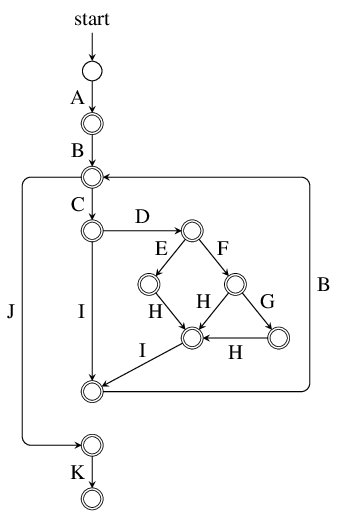This first automaton is a direct encoding of our example Control-Flow Graph (CFG). Note that each CFG edge contributes one transition to the automaton, labeled with the target node's symbol.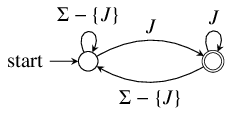This second automaton encodes the crashing requirement. Specifically, it forces accepted executions to end with CFG node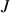.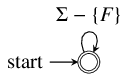The third automaton encodes the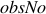constraints. That is, it forces accepted executions to not contain CFG nodes in. In this case, it ensures that no matched execution contains CFG node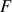.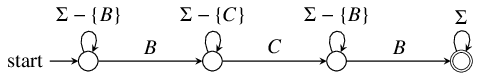The final automaton encodes an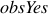constraint. For our example from the paper, there is only one such constraint. However, in the general case, we would require oneautomaton for each vector contained in. In this case, we are encoding the constraint that all matched executions must pass through CFG node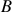, followed by CFG node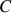, followed by another occurrence of.

### Probes to compute exeYes, exeNo, and maybe

To actually compute,, and, we first create two automata for each node in our control-flow graph,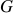. Checking the intersection of each automaton (independently) withfor emptiness indicates whether the respective node is possible and/or necessary. Here, we give the automata corresponding to probes for node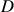.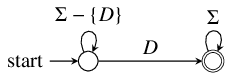The first,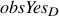corresponds to theautomaton for the single-elementvector,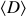. If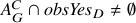, thenis possible.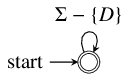The second automaton,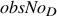corresponds to the automaton for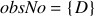. If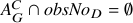, thenis necessary.

If a node,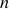is necessary, then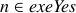. Ifis not possible, then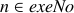. Otherwise,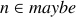.

This section provides the full formulations for the logic programs used in Section 2.2 of the paper.

### Failure Report Constraints

The failure report example described in the paper is here encoded in ASP format:

```% CFG representation
entry(a).
node(a). node(b). node(c). node(d). node(e). node(f).
node(g). node(h). node(i). node(j). node(k).

edge(a, b). edge(b, c). edge(b, j). edge(c, d). edge(c, i).
edge(d, e). edge(d, f). edge(e, h). edge(f, g). edge(f, h).
edge(g, h). edge(h, i). edge(i, b). edge(j, k).

% Observations
crash(j).
anyObsYes(b). anyObsYes(c). order(b, c). order(c, b).
obsNo(f).
```

### Background Constraints

The base encoding of the failure report consists of several rules which specify how paths through the described graph function. To do so, we introduce the concept of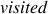(which in the encoding means any node which is visited by a possible path of execution) and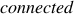(here meaning that two nodes are connected during a possible path of execution). These concepts are encoded as follows:

```0 { visited(X) } 1 :- node(X).

visited(X) :- entry(X).
visited(X) :- crash(X).
-visited(X) :- obsNo(X).
visited(X) :- anyObsYes(X).
```

Roughly, any node can potentially be; the entry and crash nodes (as well as any in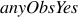) are, by definiton,. Any node observed to, specifically, not be visited (i.e., those in) are explicitly not.

```connected(X, X) :- visited(X).
connected(X, Z) :- visited(X), edge(X, Y), connected(Y, Z).
```

A node isto itself if (and only if) it is; as well, inductively, a node isto another node if it is bothand has an edge to another node which isto the specified node.

### Probes to compute exeYes, exeNo, and maybe

To actually compute,, and, we conjoin our complete set of constraints from a failure report with two probes. This section describes that process, and what SAT and UNSAT mean in each context.

#### exeYes

To definein our ASP encoding, we first define a new concept,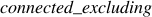, which tests if two nodes arewithout the path of execution passing through a specific third node.

```connected_excluding(X, Y, E) :-
node(E), edge(X, Y),
E != Y, E != X,
not obsNo(Y).
connected_excluding(X, Z, E) :-
connected_excluding(X, Y, E),
connected_excluding(Y, Z, E).
connected_excluding(X, X, E) :-
node(E), node(X), X != E.
```

With this concept defined, definingwithin ASP becomes straightforward:

```exeYes(X) :- crash(X).
exeYes(X) :- entry(X).
exeYes(X) :- obsYes(X).
```

The crash node, entry node, and any explicitly observed nodes are contained in.

```exeYes(X) :-
exeYes(O), crash(C), node(X),
not connected_excluding(O, C, X).

exeYes(X) :-
entry(E), exeYes(O), node(X),
not connected_excluding(E, O, X).
```

All possible paths of execution through the program must start at the entry node and end at the crash node. Any additional nodes we know to have executed–whether through explicit observation or deduction–must then have a path both from the entry and to the crash. As such, any nodes that such a path must pass through are also known to have been executed.

The known ordering of nodes during a path also figure in to the encoding:

```exeYes(X) :-
order(Y, Z), node(X),
not connected_excluding(Y, Z, X).

exeYes(X) :-
node(X),
order(A, B),
order(C, D),
A != C,
not connected_excluding(B, C, X),
not connected_excluding(D, A, X).
```

Roughly, any node that must be executed when two nodes known to have been executed in a specific order are in, as well as any which must be executed to connect multiple ordered nodes together.

#### exeNo

The calculation of membership ofis somewhat simpler:

```pospair_from(X, O) :-
connected(O, X),
connected(X, C),
crash(C),
exeYes(O).

pospair_to(X, O) :-
entry(E),
connected(E, X),
connected(X, O),
exeYes(O).

exeNo(X) :-
node(X),
exeYes(O),
not pospair_to(X, O),
not pospair_from(X, O).
```

If, for any node known to be executed, we cannot find a path from the entry to that node through a specified node and we cannot find a path from the known-executed node through the specified node to the crash, that specified node cannot have been executed.

#### maybe

If we knowand, thenis a simple choice of “none of the above”:

```maybe(X) :-
node(X),
not exeNo(X),
not exeYes(X).
```

#### Probes

The ASP encoding of the failure report allows easy direct queries of the membership of the defined sets by adding the membership of a node to a proposed set as a fact along with the encoding of the observations. For example, if we wish to directly test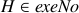, we can add exeNo(H) to the input to the ASP grounder, and then determine its truth by the SAT solver's output: a result of SAT tells us our assertion was true with UNSAT proving our assertion false.